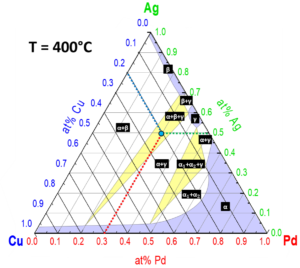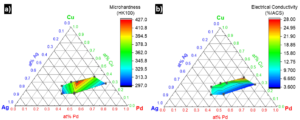# Introduction to the Pd-Ag-Cu Ternary Alloy System

#### Wade A. Jensen, Ph.D., Sr. Research Metallurgist

Many Deringer-Ney specialty alloys are composed of three or more precious metal elements. For example, many of the Paliney alloys are based on the Pd-Ag-Cu ternary system. To understand these complex systems, metallurgists use ternary phase diagrams to predict the phases present at equilibrium, as well as their elemental compositions and fractions. By varying constituent composition and thermal treatments, properties such as hardness and electrical conductivity can be engineered and optimized. Where binary phase diagrams¹ have two axes of composition and temperature, ternary diagrams can be visualized as isothermal sections with three axes of composition. The rules and learnings from the binary phase diagrams still apply, but can be easily confused due to the ternary plot’s unfamiliar nature.

The Pd-Ag-Cu ternary phase diagram at 400°C can be seen in Figure 1. Each vertex of the triangle equates to a pure element and the triangle’s edges corresponds to the binary phase diagram at the given temperature. Between each single-phase region (white) exists a two-phase region (purple) and between each two- hase regions exist a three-phase region (yellow). Many ternary phase diagrams, including this
one, were modeled via thermodynamic data and calculations and the disposition of solvus lines may not be accurate. The bulk composition (Pd30Ag20Cu50, at%) is represented by the turquoise–colored point and rests in a α + β + γ three-phase region. In this phase field, α (Ag-Pd-Cu based solid solution), β (Cu- Pd based solid solution), and γ (CuPd-based ordered phase) coexist at equilibrium.Figure 1: Annotated Pd-Ag-Cu ternary phase diagram section at 400°C. Single-phase regions are purple, two- phase regions are white, and three-phase regions are yellow. Adapted from ASM International Diagram No. 950435.

The bulk composition for this point is determined by tracing grid-parallel lines to the axes; these values
should always sum to unity. Inside of the three-phase region, the vertices denote the invariant phase composition²; meaning small variations in bulk composition only alter the phase fraction and not phase composition. The phase composition of the two-phase regions can be determined by drawing tie-lines to the solvus curves and using these points to extend lines to the axes. The phase fraction for the two-phase and three-phase region can be calculated from the diagram, but this technique will not be discussed here. As is often the case with material properties, the optimization of hardness and electrical conductivity are contradictory; strengthening Ag and Cu alloys inherently lower their ability to conduct electricity. We can observe this phenomenon in Figure 2, which depicts ternary contour plots relating the composition of age-hardened (HT Temper) Paliney alloys with microhardness and electrical conductivity. It should be noted that these alloys contain small amounts of additional solute, and these at% reflect the ratio of Pd, Ag, and Cu elements only. The trends show that certain alloys perform better for different applications, and can be selected to satisfy a diverse set of engineering constraints. For this reason, Deringer-Ney provides a number of Paliney alloys with a range of properties and precious metal content, so that customers can find a specialized product that best suit their needs.Figure 2: Ternary contour plots depicting a) microhardness and b) electrical conductivity as functions of composition. Data taken from https://www.deringerney.com/resource-library/

References:

1. W.A. Jensen, How to Interpret Binary Alloy Phase Diagrams, (2022). https://www.deringerney.com/resource-library/.
2. D.A. Porter, K.E. Easterling, 1.7 Ternary Equilibrium, in: Phase Transformations in Metals and Alloys, Second Edition, Chapman & Hall, 1997: pp. 48–52.

Share: